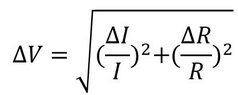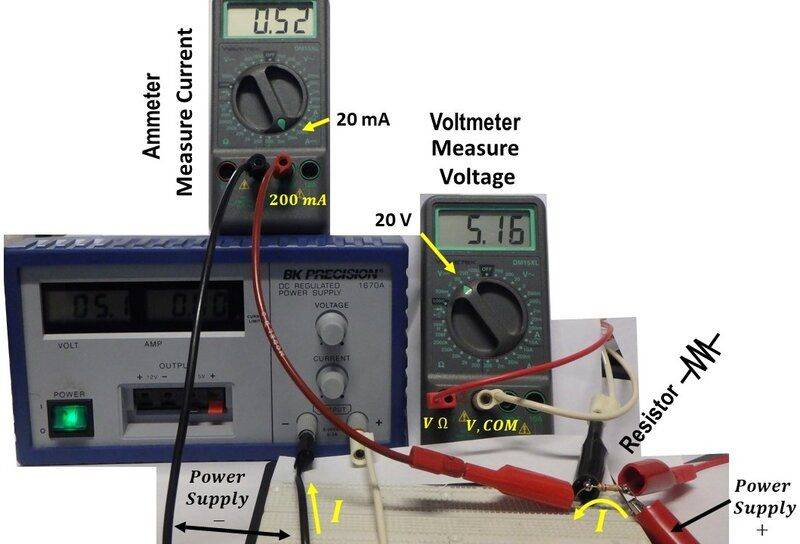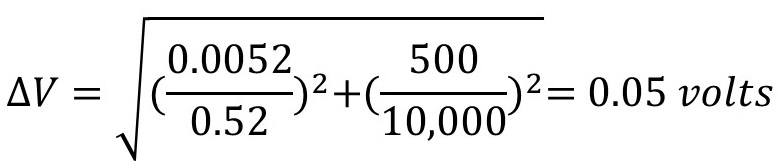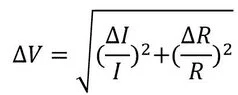# Propagation of Errors, Ohm's Law

• I
• Albertgauss

#### Albertgauss

Gold Member
TL;DR Summary
I need help in applying "Propagation of Errors" to understand Ohm's Law for error analysis
Hello,

I need help with making sure I am using instrumentation error analysis correctly through an experiment in which I verify Ohm’s Law for a simple circuit. I do have a few questions below. I calculated and measured the error two different ways and did not get the same error by both methods, so I need help in understanding what/if I did wrong.

First question: Is “Propagation of Errors” the best choice of error analysis where your measured errors are due to your measuring devices? For this example, I am going to use multimeters to measure the current/voltage through/across a resistor.

V=IR is Ohm’s Law here for the simple circuit below. I am predicting what the voltage across the resistor should be given a measured current through a measured resistance, and then measuring that voltage directly for comparison.

I believe the error I measure for the voltage across the resistor should be (since V=IR depends on its quantities through multiplication) from the current and resistance:Is this correct?

In the picture below, you can see some of my measurements and my setup.The resistor in the bottom right of the image is a 10 k-Ohm which has an error of 5%. So the uncertainty would be 500 Ohms on the resistor.

I measured a current of 0.52 mA through the resistor. According to the manual for this particular multimeter (Wavetek DM15XL) the 20mA setting has

15XL: ±(1.2% Rdg + 1 dgt) 10/15/16XL: ±(1% Rdg + 1 dgt)

Do I interpret this correctly when I say the error on the 20mA setting is 1% of 0.52mA, or .0052mA? I‘m not sure what the “+ 1 dgt” means. Something to do with “1 digit” but which digit, is my guess?

Putting these values in, the measured error on my voltage would be, as derived from the values of the resistance and current:Now, this is more uncertain (twice as much) than the uncertainty of the voltage quoted by the wavetek manual as (0.5% rdg +1 dgt), which would be 0.0258 Volts.

Which error would I would use as the actual error on the voltage, the error derived from the RI measurements (0.05 Volts) or the error published for the device in its manual upon measuring voltage directly (0.0258 Volts)?First, this is not the error in voltage. It is the relative voltage error, that is ΔV/V.

In general, if you have an instrument with a known calibration and uncertainty estimate you should use that reading. The calculated uncertainty (a better term than error) using readings of other parameters should give you a larger uncertainty since the individual uncertainties when combined must be expected to produce a larger or smaller value. The instruments for these parameters may in reality both read high or both read low thus extending the uncertainty range.

EDIT: Use the voltmeter uncertainty if its uncertainty is less than that of the combined ammeter and resistor. But keep in mind that you have two possible places to make a mistake in determining the current and resistance and only one with the voltmeter.

Last edited:
"EDIT: Use the voltmeter uncertainty if its uncertainty is less than that of the combined ammeter and resistor."

In fact, could I interpret the above to mean that, where I am interested in reporting an uncertainty in measurement, to simply quote the voltage uncertainty of the instrument that is published in its manual? If so, that would be obviously be much faster than calculating the uncertainty measurements of any equipment at all. (that is, I would not even need to calculate any uncertainty of the current and resistor in the first place in this example; just quote the error in the user's manual on the voltage and be done)

just quote the error in the user's manual on the voltage and be done

Yes, but one caveat. If the voltage measurement accuracy is critical one should verify the accuracy of the voltmeter with a known voltage reference source especially if you do not know the history of the device.

Regarding the "one digit", I believe it is the last digit. If you read 5.16 with 0.5% + 1 digit accuracy the uncertainty would be 5.16 x 0.005 +0.01 = 0.026 + 0.01 = 0.036 ≈ 0.04 or 0.8%

Sounds good. One thing I noticed, "≈ 0.04 or 0.8%" . Why did you double the 0.04? Is that to cover the +/-; that is, my voltage could be as low as 5.16-0.04 but as high as 5.16+ 0.04, for a "width" of 0.008?

Why did you double the 0.04?
The 0.04 is the uncertainty of the voltage 5.16±0.04 volts. The 0.04 is 0.8% of 5.16.

I got it! All good here. Consider question(s) answered.

•gleem